## Gnuplot

Gnuplot is a free plotting tool that can be run from the command-line or invoked by third-party applications. Despite its name, the program is not related to the GNU project. The plotting commands and the input data are read from files or stdin. The development of Gnuplot started already in 1986.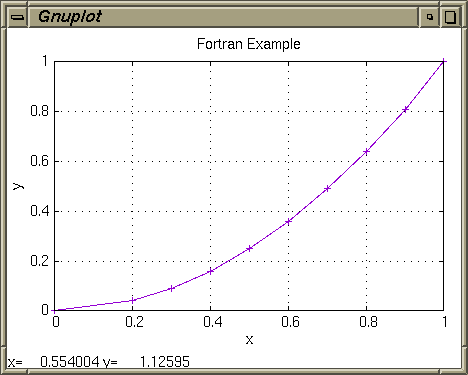Fig. 1: Gnuplot called from Fortran

Plots can either be displayed inside a window (fig. 1) or exported in various image formats:

### Command-Line Execution

The Fortran example below writes the output data to a text file `data.txt`, and then uses the intrinsic Fortran 2008 routine `execute_command_line()` to call Gnuplot. Additional Fortran modules are not required.

``````! example.f90
program main
implicit none
character(len=*), parameter :: OUT_FILE = 'data.txt' ! Output file.
character(len=*), parameter :: PLT_FILE = 'plot.plt' ! Gnuplot file.
integer,          parameter :: N        = 10         ! Number of values.

integer :: i, fu
real    :: x(N), y(N)

x(1) = 0.0
y(1) = 0.0

do i = 2, N
x(i) = 0.1 * i
y(i) = x(i)**2
end do

open (action='write', file=OUT_FILE, newunit=fu, status='replace')

do i = 1, N
write (fu, *) x(i), y(i)
end do

close (fu)

call execute_command_line('gnuplot -p ' // PLT_FILE)
end program main``````

The command-line argument `-p` lets the opened window persist. The Gnuplot commands to display the plot are stored in file `plot.plt`:

``````# plot.plt
set term x11 font "-*-helvetica-medium-r-*-*-14-*-*-*-*-*-*-*"
set title "Fortran Example"
set nokey
set grid
set xlabel "x"
set ylabel "y"
m="data.txt"
plot m using 1:2 with linespoints``````

The file will then be read by Gnuplot to perform the actual plotting:

``````\$ gfortran12 -o example example.f90
\$ ./example``````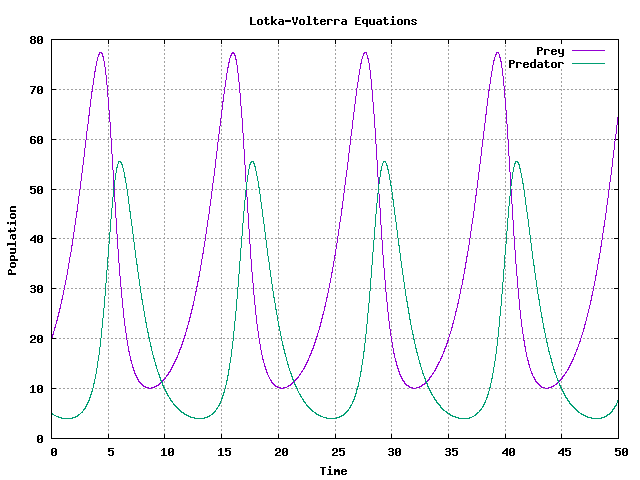Fig. 2: Output of Gnuplot as image file

### File

We can extend the approach and configure Gnuplot to save the plot to an image file instead. The next example `rk4.f90` solves the Lotka-Volterra system, a pair of non-linear ordinary differential equations that describe an ecological predator-prey model:The program implements the Runge-Kutta 4th order method to solve the equations. The results are simply printed to standard output, to be piped to file, and then plotted with Gnuplot.

``````! rk4.f90
program main
use, intrinsic :: iso_fortran_env, only: r8 => real64
implicit none
! Point estimates for model parameters taken from:
!     https://www.math.tamu.edu/~phoward/m442/modbasics.pdf
real(kind=r8), parameter :: ALPHA = 0.470_r8  !! Reproduction rate of prey.
real(kind=r8), parameter :: BETA  = 0.024_r8  !! Death rate of prey by predator.
real(kind=r8), parameter :: DELTA = 0.023_r8  !! Reproduction rate of predator by prey.
real(kind=r8), parameter :: GAMMA = 0.760_r8  !! Death rate of predator.

real(kind=r8), parameter :: T_MAX = 50.0_r8   !! Max. time.
real(kind=r8), parameter :: H     = 0.01_r8   !! Step size.
integer,       parameter :: N     = T_MAX / H !! Number of steps.

integer       :: i
real(kind=r8) :: r(2)
real(kind=r8) :: t(N), x(N), y(N)

! Steps.
t = [ (H * i, i = 1, N) ]

! Initial prey and predator population.
r = [ 20.0_r8, 5.0_r8 ]

! Solve and print results to standard output.
do i = 1, N
x(i) = r(1)
y(i) = r(2)

r = r + rk4(r, t(i), H)
print '(3(f15.8))', t(i), x(i), y(i)
end do
contains
pure function f(r, t)
!! Lotka-Volterra equations.
real(kind=r8), intent(in) :: r(2) !! Values.
real(kind=r8), intent(in) :: t    !! Step.
real(kind=r8)             :: f(2)
real(kind=r8)             :: u, v

u = r(1)
v = r(2)

f(1) =  u * (ALPHA - BETA * v)
f(2) = -v * (GAMMA - DELTA * u)
end function f

pure function rk4(r, t, h)
!! Runge-Kutta 4th order solver for function `f(r, t)`.
real(kind=r8), intent(in) :: r(2) !! Values.
real(kind=r8), intent(in) :: t    !! Step.
real(kind=r8), intent(in) :: h    !! Step size.
real(kind=r8)             :: rk4(2)
real(kind=r8)             :: k1(2), k2(2), k3(2), k4(2)

k1 = h * f(r,            t)
k2 = h * f(r + 0.5 * k1, t + 0.5 * h)
k3 = h * f(r + 0.5 * k2, t + 0.5 * h)
k4 = h * f(r + k3,       t + h)

rk4 = (k1 + (2 * k2) + (2 * k3) + k4) / 6
end function rk4
end program main``````

The Gnuplot script `plot.plt` sets the output format to PNG, and the file name to `plot.png`. The timeseries are read from file `data.txt`:

``````# plot.plt
set term png
set output "plot.png"
set title "Lotka-Volterra Equations"
set grid
set xlabel "Time"
set ylabel "Population"
plot "data.txt" using 1:2 with lines title "Prey", "data.txt" using 1:3 with lines title "Predator"``````

Compile the program source, execute the binary `rk4`, pipe the output to `data.txt`, and finally plot to `plot.png` by running the Gnuplot script `plot.plt`:

``````\$ gfortran12 -o rk4 rk4.f90
\$ ./rk4 > data.txt
\$ gnuplot -c plot.plt``````

Open `plot.png` in an image viewer (fig. 2). We can further automate the plotting step with the help of a short shell script `makeplot.sh`:

``````#!/bin/sh

set -e

./rk4 > data.txt
gnuplot -c plot.plt``````

Save the script in the same directory as the binary `rk4`, and set execution rights:

``````\$ chmod a+x makeplot.sh
\$ ./makeplot.sh``````

### Anonymous Pipe

Instead of transfering the output data to Gnuplot by file, we can feed plotting options and data values directly by connecting both applications with Unix pipes. The output of the Fortran program will become the input of Gnuplot.

The following example shows how to plot a shaded height map based on Perlin noise with Gnuplot (fig. 3). The Fortran program will simply print the height map to stdout. If we set the `dgrid3d` option followed by the correct dimensions of the matrix, Gnuplot will map a non-grid input to the internal grid format automatically. We could then output single coordinates (x, y, z) line by line instead of the matrix as a whole. Obviously, this is slower.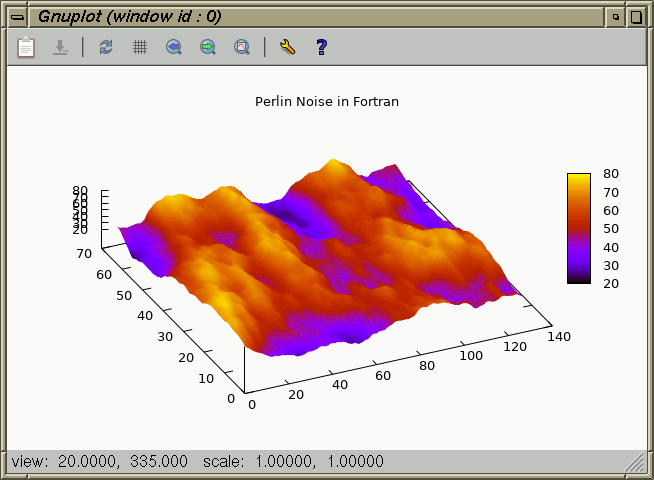Fig. 3: Perlin noise generated in Fortran and visualised by Gnuplot

The Fortran module perlin.f90 provides the basic Perlin noise implementation that is called by the example. Gnuplot will additionally interpolate the surface.

``````! matrix.f90
program main
use :: perlin
implicit none
integer, parameter :: WIDTH  = 128
integer, parameter :: HEIGHT = 64

integer :: x, y
real    :: r
real    :: matrix(WIDTH, HEIGHT)

! Set Perlin noise seed.
call random_seed()
call random_number(r)
call perlin_noise_seed(int(r * 10**6))

! Set Gnuplot options.
print '(a)', 'set nokey'                           ! Disable legend.
print '(a)', 'set cbtics scale 0'                  ! Disable colourbar tics.
print '(a)', 'set title "Perlin Noise in Fortran"' ! Set title.
print '(a)', 'set pm3d interpolate 2, 2'           ! Draw 3d data as colour map.
print '(a)', 'splot "-" matrix with pm3d'          ! Plot matrix data in 3d.

! Calculate matrix values and print them to stdout.
do y = 1, HEIGHT
do x = 1, WIDTH
matrix(x, y) = perlin_noise(real(x * .1), real(y * .1), 0.4, 8) * 100
write (*, '(f8.5, " ")', advance='no') matrix(x, y)
end do
write (*, *)
end do
end program main``````

The example can be compiled and executed with:

``````\$ gfortran12 -c perlin.f90
\$ gfortran12 -o matrix matrix.f90 perlin.o
\$ ./matrix | gnuplot -p``````

We do not need to run the example each time to display the plot. Instead, redirect the output to a file first, then pipe the file contents to Gnuplot:

``````\$ ./matrix > data.txt
\$ cat data.txt | gnuplot -p``````

### Process

On Linux and FreeBSD, Gnuplot can be executed as a sub-process, and connected to Fortran through a pipe stream, using the POSIX function popen(3). The required interface bindings to the necessary C functions are included in the fortran-unix library.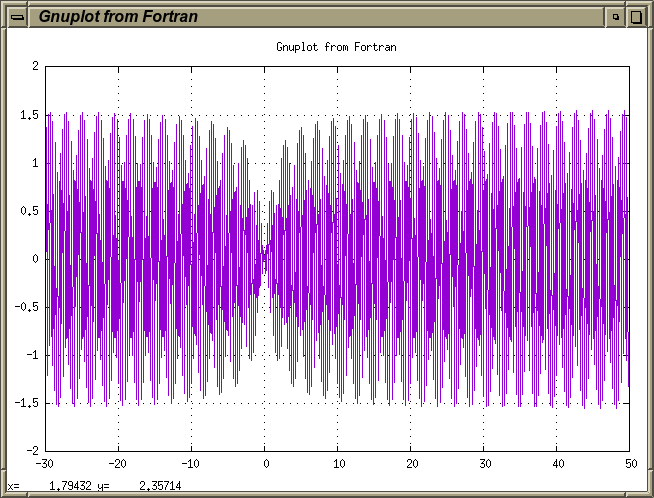Fig. 4: Calling Gnuplot through a subprocess on Unix

In this approach, we do not need to pipe the output of the Fortran program to Gnuplot from the shell. Instead, we open a pipe for writing, fork, invoke the shell, and execute Gnuplot in persistent mode, directly from Fortran, just by calling popen(3), fputs(3), and pclose(3).

Clone the fortran-unix repository and build the static library `libfortran-unix.a`:

``````\$ git clone https://github.com/interkosmos/fortran-unix
\$ cd fortran-unix/
\$ make freebsd``````

``\$ make linux``

Import the module `unix` and link the Fortran application statically against `libfortran-unix.a`.

``````! process.f90
program main
use :: unix
implicit none
character(len=*), parameter :: GNUPLOT = 'gnuplot' ! Name/path of Gnuplot binary.
integer,          parameter :: N       = 800       ! Number of values.
real,             parameter :: XSTEP   = 0.1       ! Step size.

integer :: i
real    :: x(N), y(N)

! Generate plotting data.
do i = 1, N
x(i) = -30 + ((i - 1) * XSTEP)
y(i) = sin(x(i) * 20) * atan(x(i))
end do

! Plot the data as line chart.
call plot(x, y, 'Gnuplot from Fortran')
contains
subroutine plot(x, y, title)
!! Opens pipe to Gnuplot, writes Gnuplots settings, and plots
!! X, Y data as line chart in an X11 window.
real,             intent(inout) :: x(:)  !! X values.
real,             intent(inout) :: y(:)  !! Y values.
character(len=*), intent(in)    :: title !! Plot and window title.

character(len=80) :: buffer
integer           :: i, rc
type(c_ptr)       :: ptr

! Create a pipe for writing, then fork, invoke the shell, and run Gnuplot.
ptr = c_popen(GNUPLOT // c_null_char, 'w' // c_null_char)

if (.not. c_associated(ptr)) then
print '("Error: failed to open pipe to Gnuplot")'
return
end if

! Open persistent X11 window, set window title.
call puts(ptr, 'set terminal x11 persist title "' // trim(title) // '"')

call puts(ptr, 'set title "' // trim(title) // '"') ! Plot title.
call puts(ptr, 'set grid')                          ! Enable grid.
call puts(ptr, 'set nokey')                         ! Disable legend.
call puts(ptr, 'plot "-" using 1:2 with lines')     ! Create line chart.

! Output X, Y data.
do i = 1, size(x)
write (buffer, '(2(f12.8))') x(i), y(i)
call puts(ptr, buffer)
end do

! End plot data.
call puts(ptr, 'e')

! Close the pipe.
rc = c_pclose(ptr)
end subroutine plot

subroutine puts(ptr, str)
!! Writes string to pipe, appends new line and null termination.
type(c_ptr),      intent(in) :: ptr
character(len=*), intent(in) :: str
integer                      :: rc

rc = c_fputs(trim(str) // c_new_line // c_null_char, ptr)
end subroutine puts
end program main``````

The example program has to be linked against the fortran-unix interface library:

``````\$ gfortran12 -o process process.f90 libfortran-unix.a
\$ ./process``````

Gnuplot will run persistently as an independent process, and not terminate with the Fortran program it was called from (fig. 4).

DriverDescription
`domterm`DomTerm terminal emulator with embedded SVG
`dumb`ASCII art for anything that prints text
`sixelgd`Sixel using libgd and TrueType fonts
`sixeltek`Sixel output using bitmap graphics
`tek40xx`Tektronix 4010 and others; most TEK emulators
`tek410x`Tektronix 4106, 4107, 4109 and 420X terminals
`vttek`VT-like tek40xx terminal emulator
Table 1: Selection of Gnuplot’s terminal-based drivers

### Terminal Output

One of the lesser known features of Gnuplot is the option to display plots directly in the terminal emulator by using one of the provided pseudo-graphics drivers. We can output a list of all supported drivers in the Gnuplot interpreter:

``````\$ gnuplot
gnuplot> set term

Available terminal types:
cairolatex  LaTeX picture environment using graphicx package and Cairo backend
canvas  HTML Canvas object
cgm  Computer Graphics Metafile
context  ConTeXt with MetaFun (for PDF documents)
domterm  DomTerm terminal emulator with embedded SVG
dpu414  Seiko DPU-414 thermal printer [small medium large]
dumb  ascii art for anything that prints text
[…]``````

Or, run `echo "set term" | gnuplot` in the terminal. The backends `sixelgd` and `sixeltek` require a Sixel-compatible terminal, such as xterm(1) or mlterm(1) on Unix (table 1). Terminal emulators that do not support the format will ignore any Sixel data. XTerm has to be run in VT340 mode to enable Sixel graphics:

``\$ xterm -ti vt340``

Add the following lines to your `~/.Xdefaults` to select the mode by default:

``````xterm*decTerminalID: vt340
xterm*numColorRegisters: 256``````

Or, start XTerm in Tektronix mode by running `xterm -t` instead. The environment variable `TERM` should be set to `xterm`.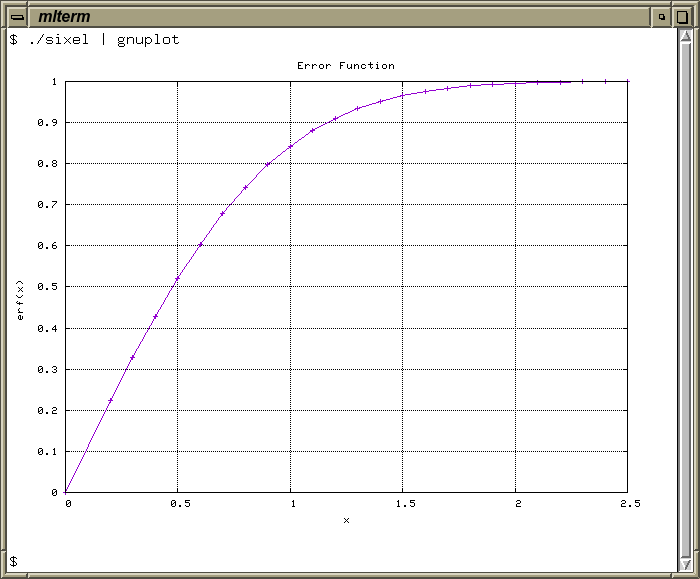Fig. 5: Plotting directly to mlterm(1) through the Gnuplot Sixel backend

The example program `sixel.f90` prints the results of the intrinsic error functionand all necessary Gnuplot options to stdout. We can change the backend in parameter `TERM`.

``````! sixel.f90
program main
implicit none
character(len=*), parameter :: TERM   = 'sixeltek'
character(len=*), parameter :: TITLE  = 'Error Function'
character(len=*), parameter :: XLABEL = 'x'
character(len=*), parameter :: YLABEL = 'erf(x)'
integer,          parameter :: N      = 25

real    :: x(N), y(N)
integer :: i

x(1) = 0.0
y(1) = 0.0

do i = 2, N
x(i) = 0.1 * i
y(i) = erf(x(i))
end do

print '("set term ", a)', TERM                    ! Set backend.
print '("set nokey")'                             ! Disable legend.
print '("set grid")'                              ! Enable grid.
print '("set title ''", a, "''")', TITLE          ! Set plot title.
print '("set xlabel ''", a, "''")', XLABEL        ! Set x axis label.
print '("set ylabel ''", a, "''")', YLABEL        ! Set y axis label.
print '("plot ''-'' using 1:2 with linespoints")' ! Plot following data as line graph.

do i = 1, N
print '(f12.5, ",", f12.5)', x(i), y(i)
end do
end program main``````

Single quotes `'` in the format specifiers have to be escaped as `''`. Simply pipe the output of the program to Gnuplot. The result is shown immediately (fig. 5):

``````\$ gfortran12 -o sixel sixel.f90
\$ ./sixel | gnuplot``````

Optionally, we may save the Sixel image to file:

``````\$ ./sixel | gnuplot > plot.six
\$ cat plot.six``````

The library libsixel provides encoders and decoders for the Sixel format which can be invoked from the command-line.

### Fortran Libraries

A selection of Gnuplot libraries is available for Fortran:

• GNUFOR: A Fortran 90 interface for Gnuplot with data and command file output. Gnuplot is then called to run the plot script.
• GNUFOR2: An interface based on GNUFOR.
• Gnuplotfortran: A Fortran 95 interface that requires fortranposix.
• ogpf: An object-oriented interface to Gnuplot for Fortran 2003.